# How to Ace the Indeed Excel Assessment

Indeed is a popular job searching site which offers job seekers the opportunity to take assessments to prove they have certain skills. One such assessment is for Microsoft Excel. Job seekers can take the Excel assessment and (if they score well) can display the results on their Indeed profiles for companies to see. This may make the job seeker a more desirable candidate to prospective companies, especially for jobs which require Microsoft Excel skills.

Many job candidates wonder how to prepare for such an assessment. After all, if you are going to display the results on your Indeed profile, you want to make sure you put your best foot forward. This article explains how the test is structured, how to prepare, and provides some example practice problems with explanations that cover topics that might be on the assessment. Read on to learn how to score Expert on the Indeed Excel Assessment!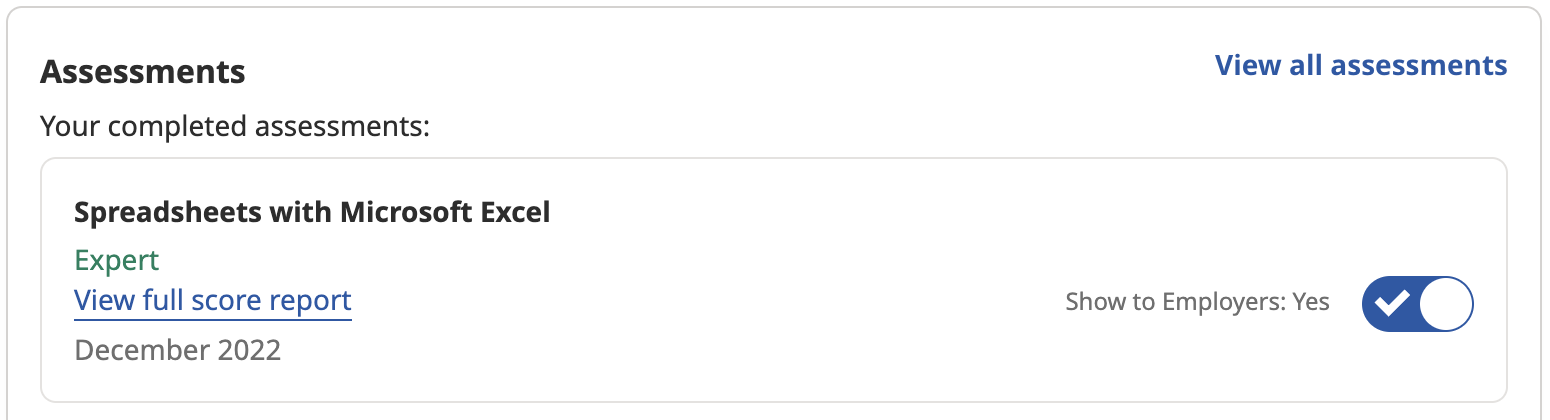## Indeed Microsoft Excel Assessment Format

The Indeed Excel assessment is a quick test that takes most people about ten minutes to complete. It comprises approximately fifteen multiple choice questions regarding formatting, Excel functions, and overall basic Excel knowledge. Each question is timed, and is typically a minute and a half to two minutes long.

After completing the assessment, you will receive one of five scores:

• Expert
• Highly Proficient
• Proficient
• Familiar
• Completed

As you may have guessed, Expert is the best score. Completed is the worst score, and simply means you completed the assessment. You will receive a score of N/A if you do not complete the assessment.

## Indeed Excel Assessment Topics

The following topics were covered by the assessment in December 2022, but this is subject to change depending on how Indeed decides to administer the assessment.

• Worksheets and referencing cells in other sheets
• MIN and MAX
• COUNTIF and COUNTIFS
• Formatting (bold, center text, fill color, etc.)
• CONCAT
• Absolute Reference
• IF

In our experience, the Indeed Excel assessment does not cover more advanced topics like pivot tables or VBA.

## Indeed Excel Assessment Practice Questions and Answers

The following practice problems will prepare you for the format and topics covered by the assessment. Note again that these were the topics when we took the assessment, but Indeed may change the topics and questions over time.

### Creating Worksheets and Referencing Cells in Other Worksheets

#### Question 1: What would clicking the + sign do in the image below?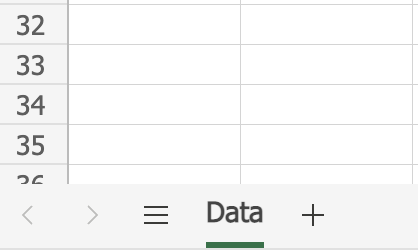A) Create a new worksheet
B) Create a new formula in the worksheet
C) Add the numbers in the worksheet
D) Create a column in the worksheet
E) Create a row in the worksheet

The tabs along the bottom represent different worksheets, and clicking the + sign creates a new one.

#### Question 2: When writing a formula in your new worksheet, how would you reference cell C5 in the Data worksheet (see image above)?

A) `C5`
B) `"Data"C5`
C) `Data\$C5`
D) `Data!C5`
E) `'Data'C5`

To reference a cell in another worksheet, an exclamation mark (!) separates the name of the worksheet and the cell reference. Note that if the worksheet name contained a space then the worksheet name must go inside single quotes. For example, if the worksheet was named Employee Data, then the cell reference would be `'Employee Data'!C5`.

For more practice, take the lesson on worksheets!

### MIN and MAX Functions

#### Question 3: What formula would return a value of 6?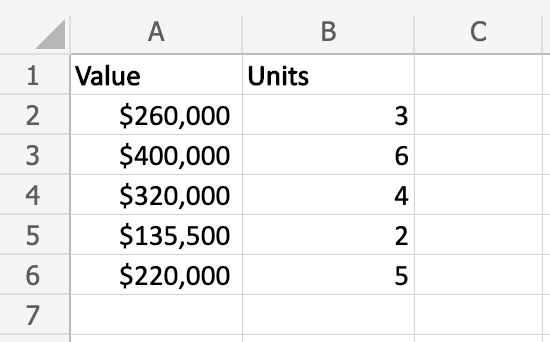A) `=CEILING(B2:B6)`
B) `=MAX(B2:B6)`
C) `=TOP(B2:B6)`
D) `=BIGGEST(B2:B6)`
E) `=HIGH(B2:B6)`

The MAX function returns the maximum value from the range of cells specified. 6 is the maximum value in the range B2:B6.

#### Question 4: What formula would return a value of \$135,500 (see image above)?

A) `=FLOOR(A2:A6)`
B) `=MIN(A2:A6)`
C) `=BOTTOM(A2:A6)`
D) `=SMALLEST(A2:A6)`
E) `=LOW(A2:A6)`

The MIN function returns the minimum value from the range of cells specified. \$135,500 is the minimum value in the range A2:A6.

For more practice, try our interactive lessons on min, max, large, and small!

### COUNTIF and COUNTIFS Function

#### Question 5: What would be returned by the formula `=COUNTIF(A:A, "*st")`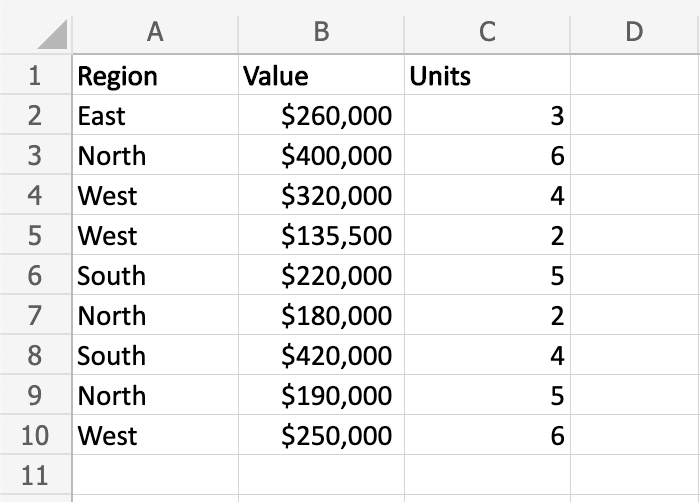A) 0
B) 1
C) 2
D) 3
E) 4

This question is asking what the result of the formula `=COUNTIF(A:A, "*st")` would be. This is essentially asking how many values in column A end with the text "st". A2, A4, A5, and A10 all end with the value "st" so the answer is 4.

#### Question 6: What would be returned by the formula `=COUNTIFS(A:A, "West", C:C, 4)` (see image above)?

A) 0
B) 1
C) 2
D) 3
E) 4

This question is asking how many rows have a region value of "West" and have a Units value of 4. There is only one row that satisfies both of these conditions, so the answer would be B.

To answer these questions, you need to know the COUNTIF and COUNTIFS functions and also how wildcards work in Excel. For more information try our lessons on the COUNTIF function and the COUNTIFS function, and see our article on wildcards.

### Formatting

#### Question 7: Which tools would you use to change the format of the headings as pictured (select all that apply)?

##### Before:##### After: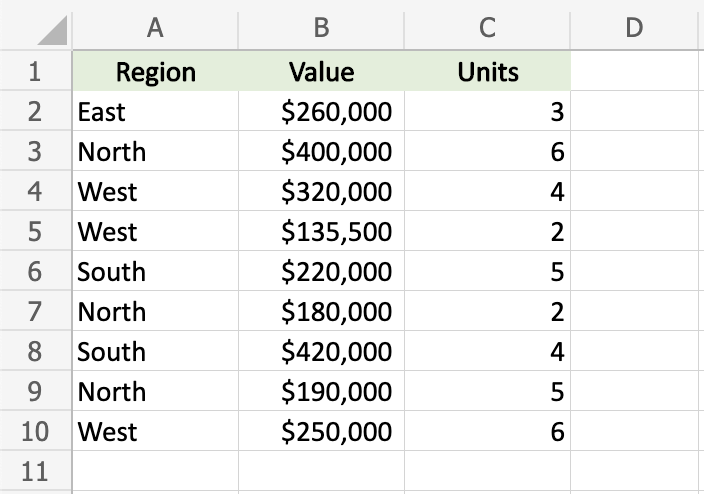A)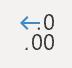B)C)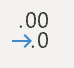D)E)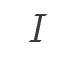This question asks you to identify all of the Excel formatting tools that would yield a result that looks like the second image. The changes made to the header include centering the text and changing the background color, thus the correct answer is to choose these two tools.

#### Question 8: Which tools would you use to change the format of the Units column as pictured (select all that apply)?

##### Before:##### After: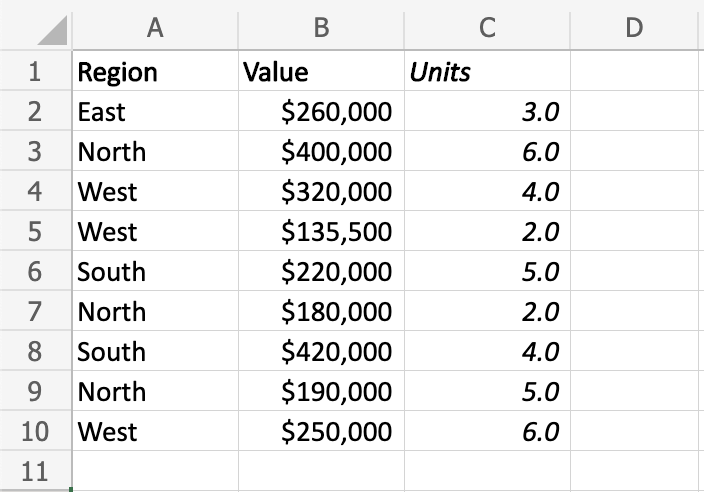A)B)C)D)E)This question asks you to identify all of the formatting tools that would yield a result that looks like the second image. The changes to the Units column include italics and adding one decimal place, thus the correct answer is to choose these two tools.

### CONCAT Function

#### Question 9: Which formula would return the text string "East Region"?A) `=RIGHT(A2, A1)`
B) `=LEFT(A2, A1)`
C) `=TEXTJOIN(A2, A1)`
D) `=CONCAT(A2, A1)`
E) `=CONCAT(A2, " ", A1)`

The CONCAT function joins all the arguments together to form a single text string. Note that there is a space between the words "East" and "Region" in the result. Therefore, option E is the answer because it concatenates the value in cell A2, then a space, then the value in cell A1. Note that CONCAT and CONCATENATE is interchangeable in most versions of Excel, and the Indeed assessment may use either form.

For more practice, try our lesson on the CONCAT function!

### Absolute Reference

#### Question 10: If the formula in cell D2 was copied down to cells D3, D4, and D5, what would appear in these three cells?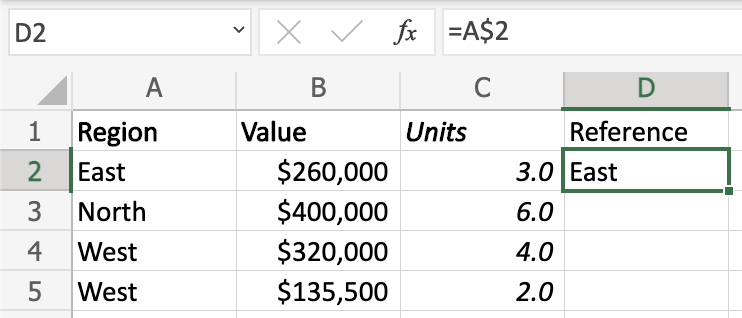A) East, East, East
B) North, West, West
C) East, North, West
D) North, North, North
E) West, West, West

Because the row number in the cell reference is anchored with the dollar sign (\$), this is an absolute reference to row 2. Because the formula is copied downward, it will always reference cell A2, resulting in East, East, East in cells D3, D4, and D5.

### IF Function

#### Question 11: Which formula would return a result of "Yes"?A) `=IF(A2="East")`
B) `=IF(A2="East", "No", "Yes")`
C) `=IF(A2="East", "Yes", "No")`
D) `=IF(C2>2, "No", "Yes")`
E) `=IF(C2=2, "Yes", "No")`

If the first argument is true, then the IF function will return the second argument. Otherwise it will return the third argument. Because A2 is equal to "East" the function will return the value "Yes" so C is the correct answer.

For more information, try our lesson on the IF function in Excel.

### Miscellaneous

#### Question 12: What is the operator to multiply in Excel?

A) /
B) *
C) +
D) -
E) #

You multiply two numerical values in Excel using the asterisk (*).

#### Question 13: How would you sort values in Excel to get an alphabetical list ordered from A to Z?

A) Ascending
B) Descending
C) Equal
D) Greater than
E) Less than

Ascending order is from smallest to largest: 0-9 or A-Z. You can remember this because "Ascending" starts with an A, just like sorting A-Z!

A) Ctrl + B
B) Ctrl + X
C) Ctrl + C
D) Ctrl + V
E) Ctrl + P